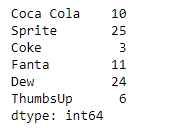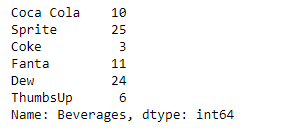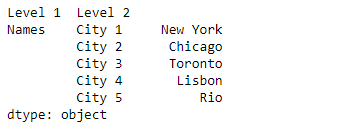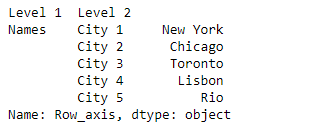Skip to content
Related Articles
Python | Pandas Series.rename()
• Last Updated : 14 Oct, 2019

Pandas series is a One-dimensional ndarray with axis labels. The labels need not be unique but must be a hashable type. The object supports both integer- and label-based indexing and provides a host of methods for performing operations involving the index.

Pandas` Series.rename()` function is used to alter Series index labels or name for the given Series object.

Syntax: Series.rename(index=None, **kwargs)

Parameter :
index : dict-like or functions are transformations to apply to the index
copy : Also copy underlying data
inplace : Whether to return a new Series. If True then value of copy is ignored.
level : In case of a MultiIndex, only rename labels in the specified level.

Returns : Series, DataFrame, or None

Example #1: Use `Series.rename()` function to rename the name of the given Series object.

 `# importing pandas as pd``import` `pandas as pd`` ` `# Creating the Series``sr ``=` `pd.Series([``10``, ``25``, ``3``, ``11``, ``24``, ``6``])`` ` `# Create the Index``index_ ``=` `[``'Coca Cola'``, ``'Sprite'``, ``'Coke'``, ``'Fanta'``, ``'Dew'``, ``'ThumbsUp'``]`` ` `# set the index``sr.index ``=` `index_`` ` `# Print the series``print``(sr)`

Output :Now we will use `Series.rename()` function to rename the name of the given series object.

 `# rename the series``result ``=` `sr.rename(``'Beverages'``)`` ` `# Print the result``print``(result)`

Output :As we can see in the output, the `Series.rename()` function has successfully renamed the given series object.

Example #2: Use `Series.rename()` function to rename the MultiIndex axis of the given Series object.

 `# importing pandas as pd``import` `pandas as pd`` ` `# Creating the Series``sr ``=` `pd.Series([``'New York'``, ``'Chicago'``, ``'Toronto'``, ``'Lisbon'``, ``'Rio'``])`` ` `# Create the MultiIndex``index_ ``=` `pd.MultiIndex.from_product([[``'Names'``], [``'City 1'``, ``'City 2'``, ``'City 3'``, ``'City 4'``, ``'City 5'``]],``                                                                      ``names ``=``[``'Level 1'``, ``'Level 2'``])`` ` `# set the index``sr.index ``=` `index_`` ` `# Print the series``print``(sr)`

Output :Now we will use `Series.rename()` function to rename the 0th level of the given series object.

 `# rename the 0th level``result ``=` `sr.rename(level ``=` `0``, index ``=` `'Row_axis'``)`` ` `# Print the result``print``(result)`

Output :As we can see in the output, the `Series.rename()` function has successfully renamed the 0th level of the given series object.

Attention geek! Strengthen your foundations with the Python Programming Foundation Course and learn the basics.

To begin with, your interview preparations Enhance your Data Structures concepts with the Python DS Course. And to begin with your Machine Learning Journey, join the Machine Learning – Basic Level Course

My Personal Notes arrow_drop_up